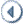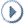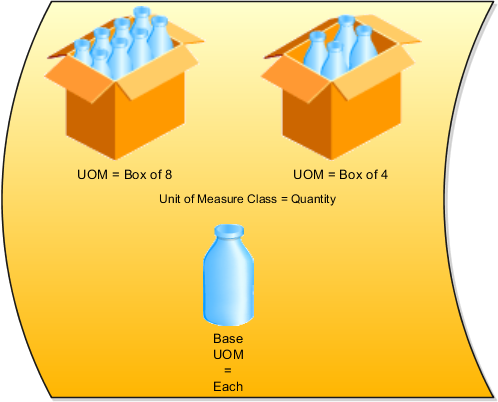Browser version scriptSkip Headers

 Oracle® Fusion Applications Product Information Management Implementation Guide 11g Release 1 (11.1.1.5.0) Part Number E20387-01ContentsPreviousNext

3 Manage Units of Measure

This chapter contains the following:

Units of Measure, Unit of Measure Classes, and Base Units of Measure: How They Fit Together

Assigning Base Units of Measure to Unit of Measure Classes: Examples

Defining Unit of Measure Standard Conversions: Examples

FAQs for Manage Units of Measure

Units of Measure, Unit of Measure Classes, and Base Units of Measure: How They Fit Together

Define units of measure, unit of measure classes, and base units of measure for tracking, moving, storing, and counting items.

The Quantity unit of measure class contains the units of measure Box of 8, Box of 4, and Each. The unit of measure Each is assigned as the base unit of measure.Unit of Measure Classes

Unit of measure classes represent groups of units of measure with similar characteristics such as area, weight, or volume.

Units of Measure

Units of measure are used by a variety of functions and transactions to express the quantity of items. Each unit of measure you define must belong to a unit of measure class.

Base Units of Measure

Each unit of measure class has a base unit of measure. The base unit of measure is used to perform conversions between units of measure in the class. For this reason, the base unit of measure should be representative of the other units of measure in the class, and should generally be one of the smaller units. For example, you could use CU (cubic feet) as the base unit of measure for a unit of measure class called Volume.

Assigning Base Units of Measure to Unit of Measure Classes: Examples

Each unit of measure class must have a base unit of measure.

Scenario

This table lists examples of unit of measure classes, the units of measure in each unit of measure class, and the unit of measure assigned as the base unit of measure for each unit of measure class. Note that each base unit of measure is the smallest unit of measure in its unit of measure class.

Unit of Measure Class

Units of Measure

Base Unit of Measure

Quantity

dozen

box

each

each

Weight

pound

kilogram

gram

gram

Time

hour

minute

second

second

Volume

cubic feet

cubic centimeters

cubic inches

cubic inches

Defining Unit of Measure Standard Conversions: Examples

A unit of measure standard conversion specifies the conversion factor by which the unit of measure is equivalent to the base unit of measure.

Scenario

This table lists examples of unit of measure classes, one unit of measure included in each class, the base unit of measure for the unit of measure class, and the conversion factor defined for the unit of measure.

Unit of Measure Class

Unit of Measure

Base Unit of Measure

Conversion Factor

Quantity

dozen

each

12

(1 dozen = 12 each)

Weight

pound

gram

454

(1 pound = 454 grams)

Time

minute

second

60

(1 minute = 60 seconds)

What's a unit of measure standard conversion?

A unit of measure standard conversion defines the conversion factor by which the unit of measure is equivalent to the base unit of measure that you defined for the unit of measure class. Defining a unit of measure standard conversion allows you to perform transactions in units other than the primary unit of measure of the item being transacted. The standard unit of measure conversion is used for an item if an item-specific unit of measure conversion has not been defined.

What's a UOM interclass conversion?

A UOM interclass conversion defines the conversion between the source base unit of measure ("From Base UOM") in one unit of measure class ("From Class") and the destination base unit of measure ("To Base UOM") in a different unit of measure class ("To Class").

For example, the item is gasoline. The From Base UOM (of the From Class called "volume") is liters. The To Base UOM (of the To Class called "quantity") is Barrels. The conversion is 158.76 liters (volume) to 1 barrel of oil (quantity).

What's a UOM intraclass conversion?

A UOM intraclass conversion specifies the conversion between a unit of measure (the "From UOM") and the base unit of measure of the same class.

For example, the item is soda pop. The unit of measure class is Quantity. The From UOM is Case (CS). The base unit of measure is Each (EA). The conversion is 24, to specify that 1 CS = 24 EA.